Symmetry Definition

Symmetry
Serial order wise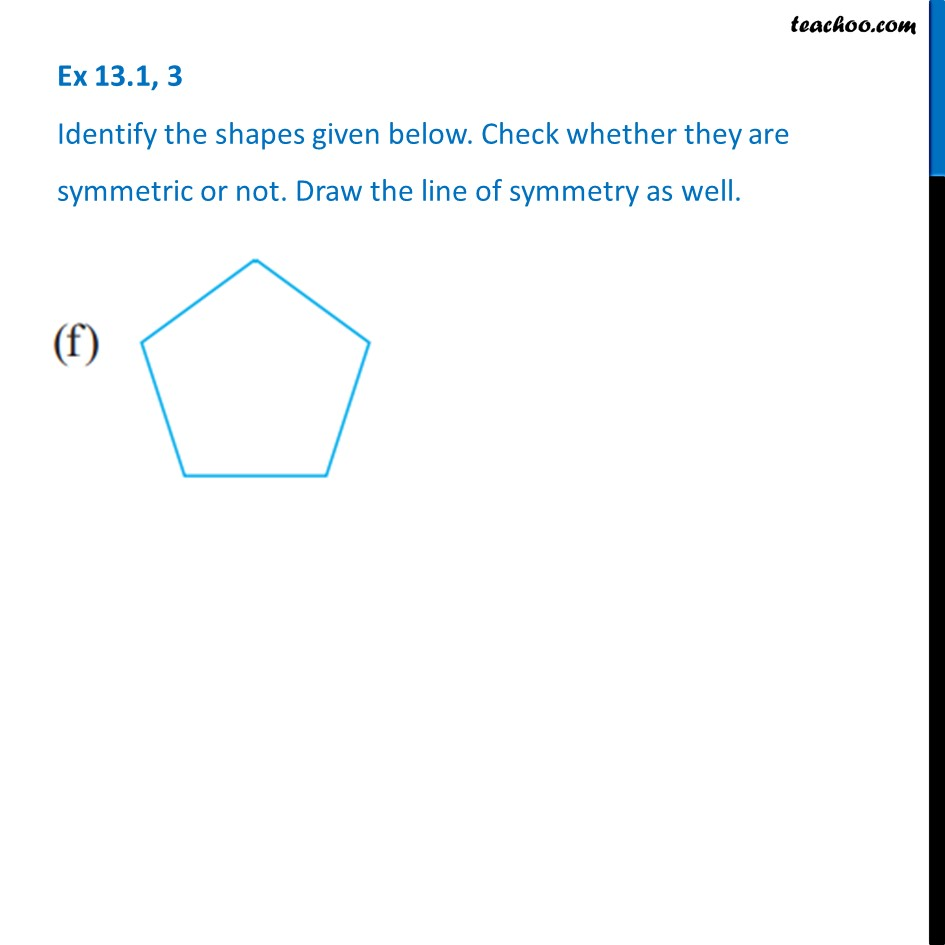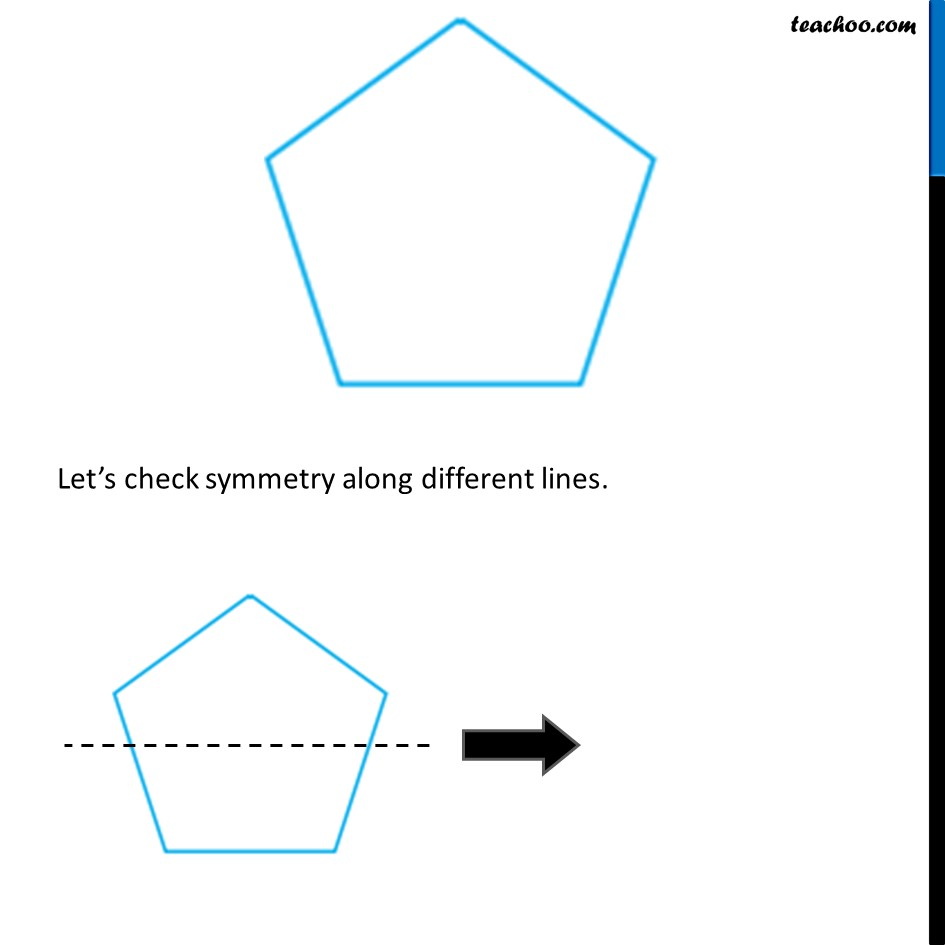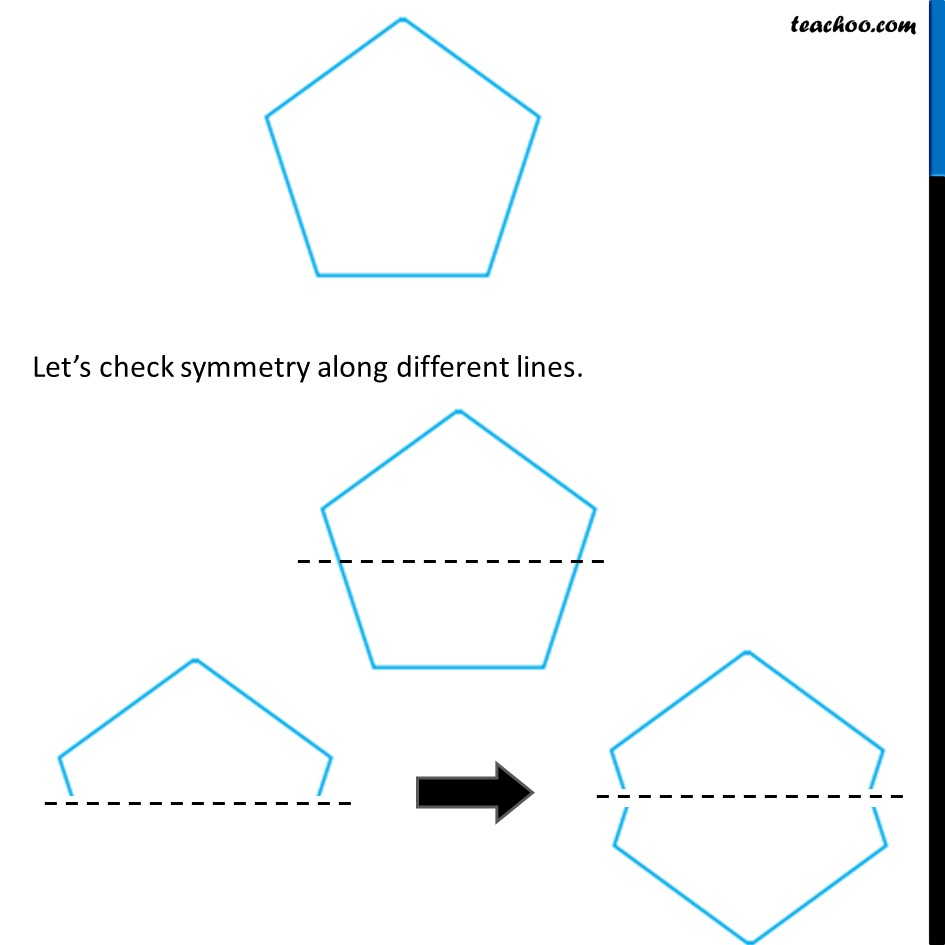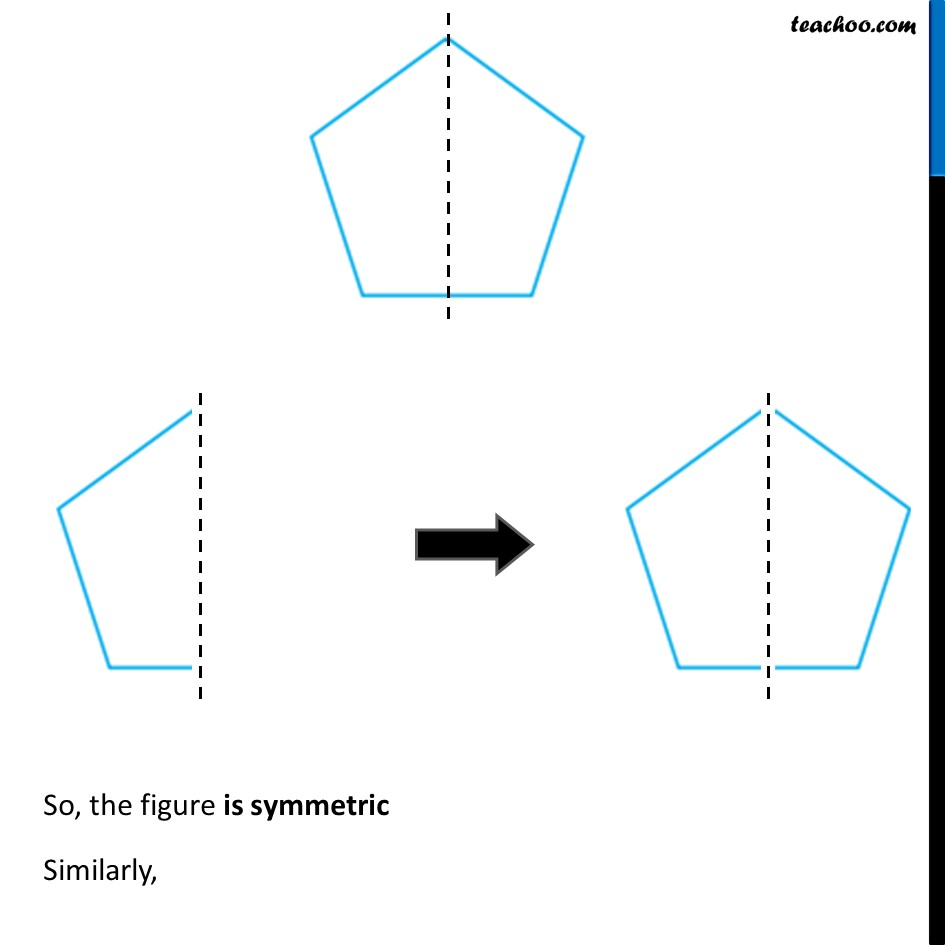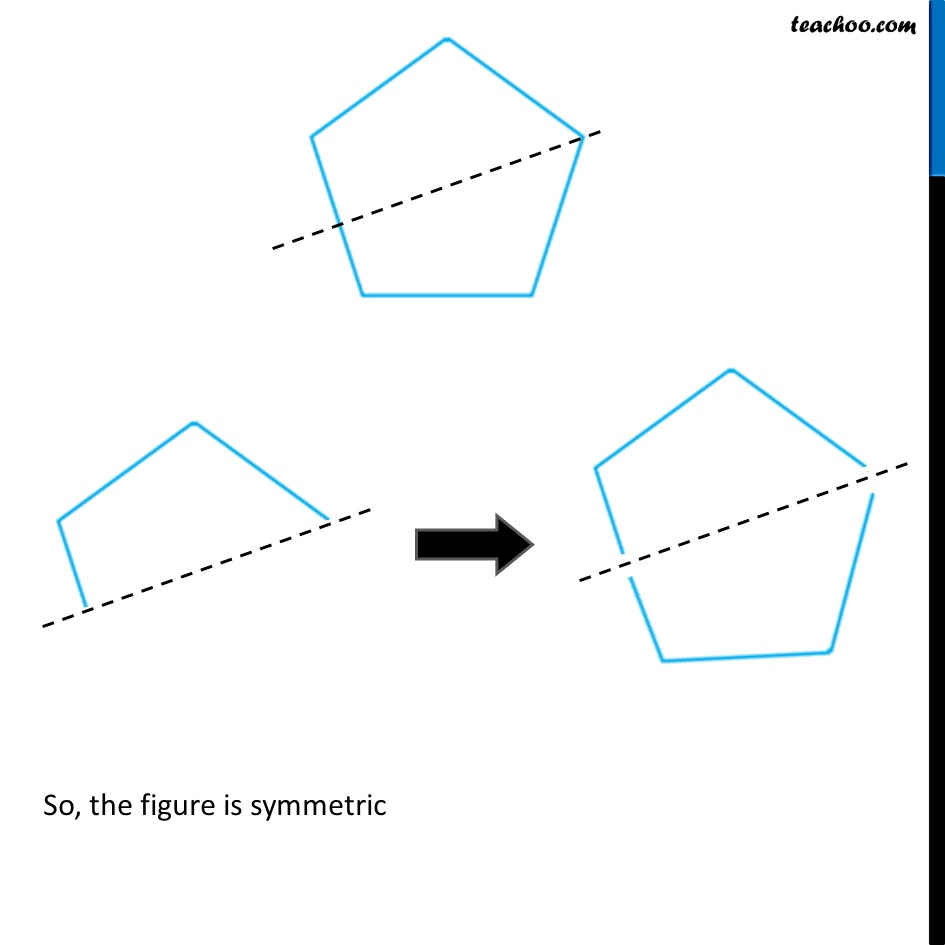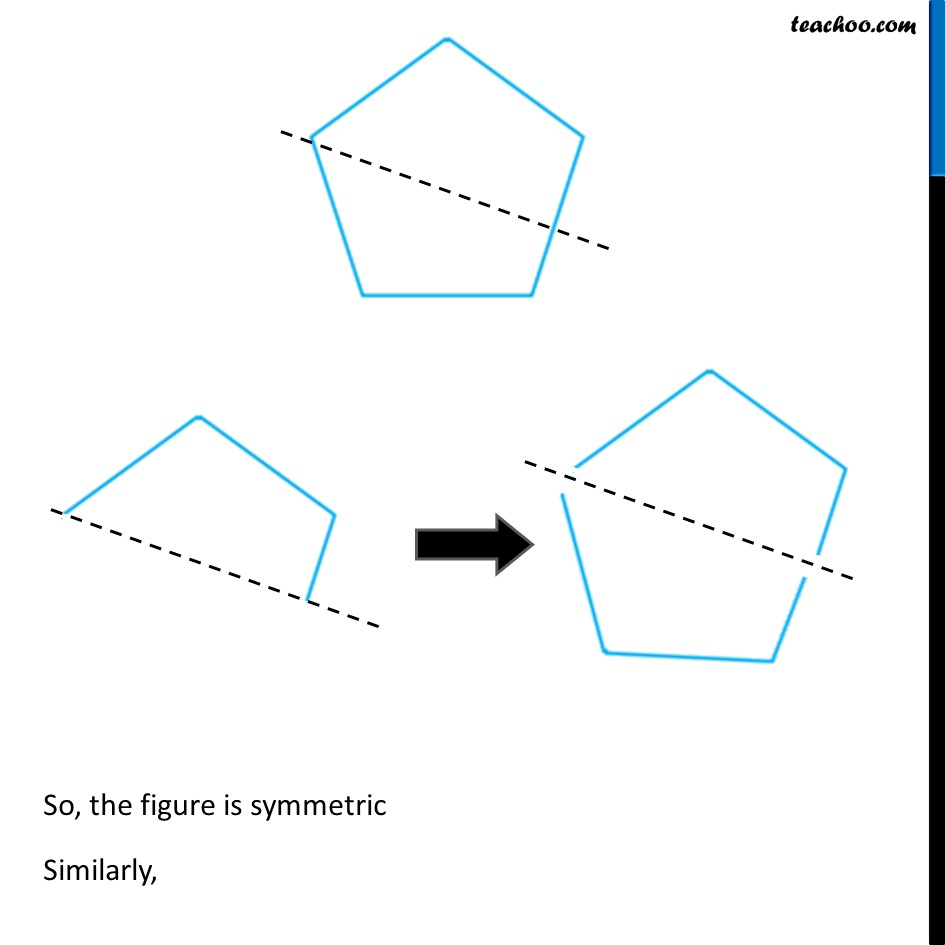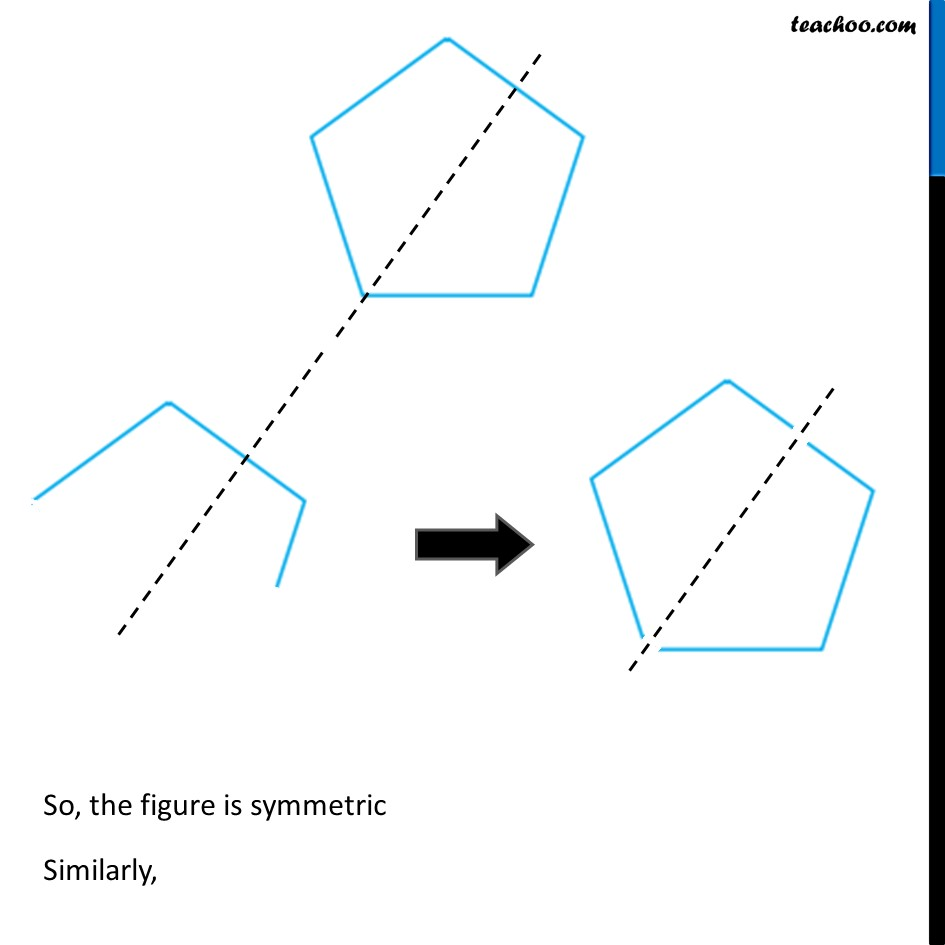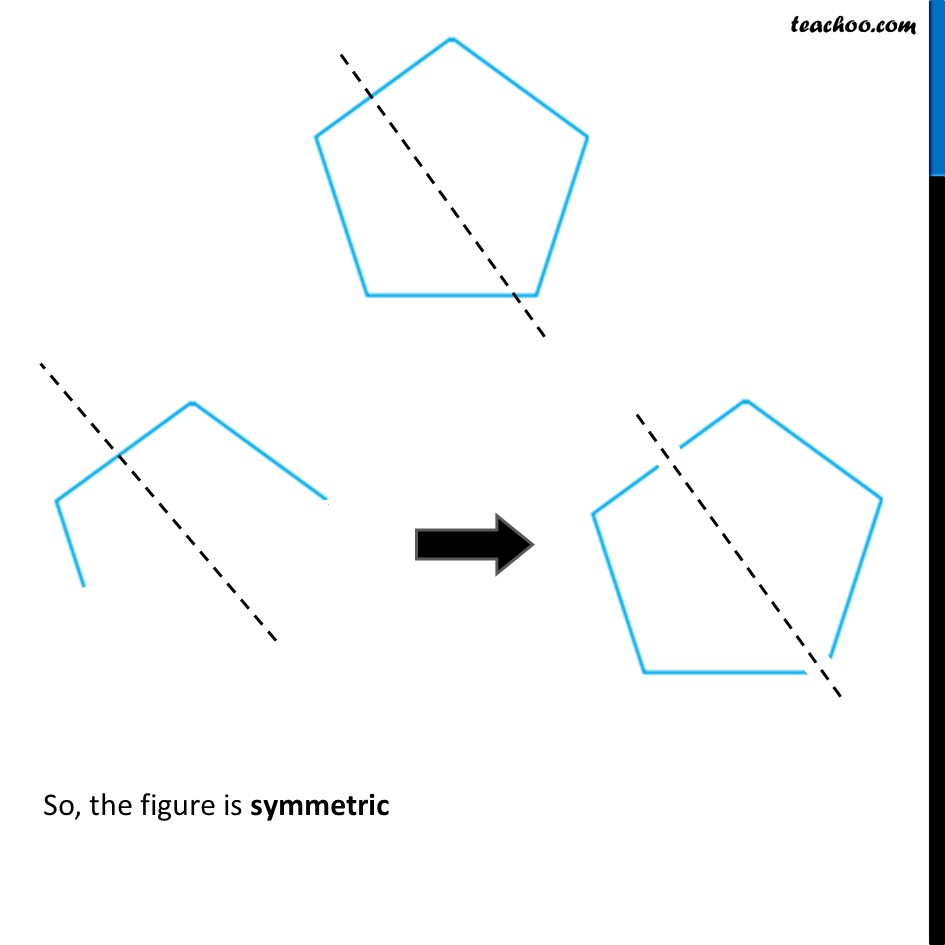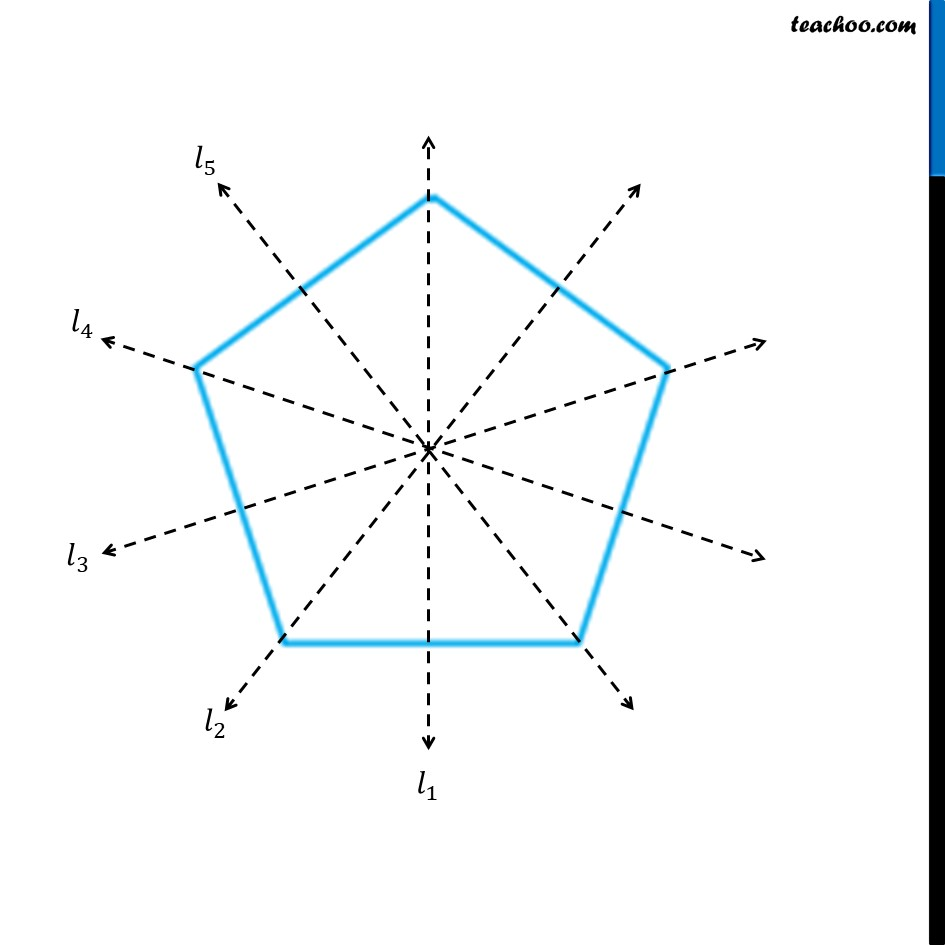Learn in your speed, with individual attention - Teachoo Maths 1-on-1 Class

### Transcript

Question 3 Identify the shapes given below. Check whether they are symmetric or not. Draw the line of symmetry as well.Let’s check symmetry along different lines. Let’s check symmetry along different lines. So, the figure is symmetric Similarly, So, the figure is symmetric So, the figure is symmetric Similarly, So, the figure is symmetric Similarly, So, the figure is symmetric